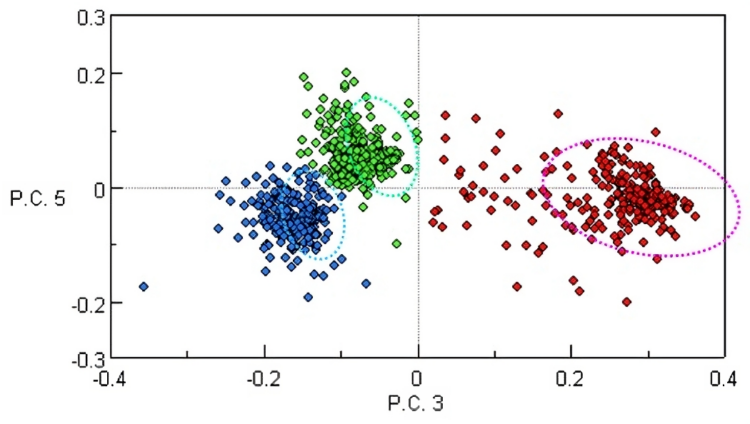IRT

# Chemometrics

Chemometrics is a statistical method that can be used to analyze spectral data by comparing unknown variables to a set of known or referenced data. Quantitative and qualitative analysis can be performed using five multivariate algorithms, including Classical Least Square (CLS), Principal Components Regression (PCR), Partial Least Square (PLS), Principal Components Analysis (PCA), and Multivariate Curve Resolution (MCR). In FTIR data analysis, chemometrics is used to determine the amount of components in a mixture.

## Quantitative Chemometrics

#### Classical Least Square (CLS)

Classical Least Square (CLS) is a prediction model that enables the determination of the concentration of unknown components based on the concentration of known multicomponent systems, assuming that the system can be represented by a linear matrix. The matrix sequence for CLS is based on the Beer-Lambert law,

Abs = C× k (C: concentration, k: absorptivity coefficient) + errors.

A calibration model is created to calculate the correlation coefficient for each component’s horizontal axis from the standard spectrum for which the multiple component concentrations are known. Calculations based on this method are very fast, but not recommended for mixtures of constituents that interact.

#### Inverse Least Square (ILS)

Inverse Least Square (ILS) uses a variation of the CLS method, where each concentration is a function of the absorbance at a series of given wavelengths. While this calculation is fast and allows for the quantification of more complex mixtures, wavelength selection can be difficult and time consuming since multiple wavelengths must be used to accurately describe each known component in the mixture. Several samples are needed for a more accurate calibration.

#### Principal Component Regression (PCR)

Principal Component Regression (PCR) is a method that combines Principal Component Analysis with Inverse Least Squares regression to create a quantitative model for complex samples. Instead of being based on Beer’s law with the aim to calculate the absorptivity coefficients for the constituents of interest from a regression of the concentration, PCR regresses concentrations on PCA scores.

#### Partial Least Square (PLS)

Partial Least Square (PLS) is a quantitative spectral decomposition technique that uses the concentration data during the decomposition of the spectral matrix, directly correlating the concentration data to the spectral data. The variations in concentration are taken into account and related to the variations in the spectral data. This form of regression leads to components with larger concentrations being weighted more heavily than components with smaller concentrations.

## Qualitative Chemometrics

#### Principal Component Analysis (PCA)

Principal Component Analysis (PCA) is a method used to reduce data to set variables, principal components, and scores and loadings based on data distribution. Each principal component calculated contains information on the variation of the data; scores are used to classify the data, and loading provides an indication of the importance of components, allowing the clustering of the scores.#### Multivariate Curve Resolution (MCR)

In the case of Multivariate Curve Resolution (MCR), the standard sample spectra are not known, only the matrix of spectra. Therefore, PCA is performed on multiple spectra, and principal component spectra are then calculated. Different components can be found in an unknown sample. Below, a cross-section of a multilayer film was imaged using MCR with a FTIR microscope, the results demonstrated that the sample consists of four components.